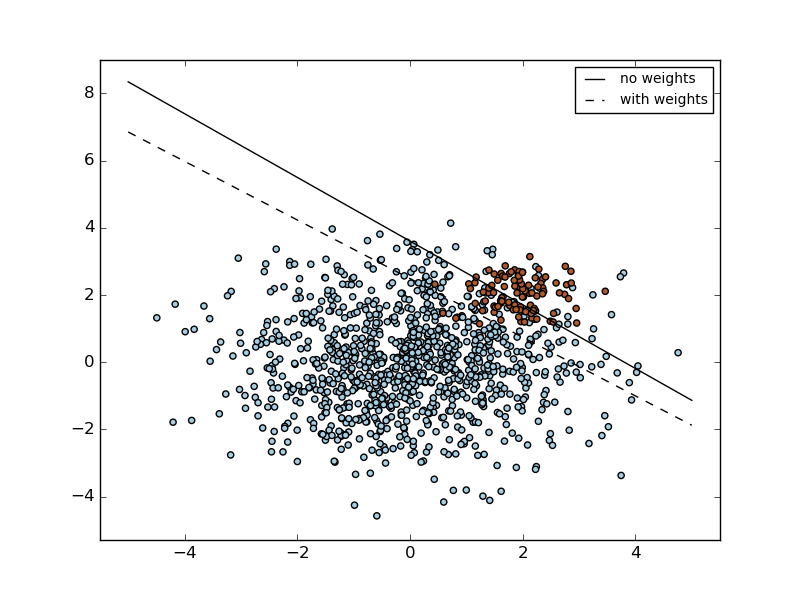# SVM: Separating hyperplane for unbalanced classes¶

Find the optimal separating hyperplane using an SVC for classes that are unbalanced.

We first find the separating plane with a plain SVC and then plot (dashed) the separating hyperplane with automatically correction for unbalanced classes.

Note

This example will also work by replacing `SVC(kernel="linear")` with `SGDClassifier(loss="hinge")`. Setting the `loss` parameter of the `SGDClassifier` equal to `hinge` will yield behaviour such as that of a SVC with a linear kernel.

For example try instead of the `SVC`:

```clf = SGDClassifier(n_iter=100, alpha=0.01)
```Python source code: `plot_separating_hyperplane_unbalanced.py`

```print(__doc__)

import numpy as np
import matplotlib.pyplot as plt
from sklearn import svm
#from sklearn.linear_model import SGDClassifier

# we create 40 separable points
rng = np.random.RandomState(0)
n_samples_1 = 1000
n_samples_2 = 100
X = np.r_[1.5 * rng.randn(n_samples_1, 2),
0.5 * rng.randn(n_samples_2, 2) + [2, 2]]
y =  * (n_samples_1) +  * (n_samples_2)

# fit the model and get the separating hyperplane
clf = svm.SVC(kernel='linear', C=1.0)
clf.fit(X, y)

w = clf.coef_
a = -w / w
xx = np.linspace(-5, 5)
yy = a * xx - clf.intercept_ / w

# get the separating hyperplane using weighted classes
wclf = svm.SVC(kernel='linear', class_weight={1: 10})
wclf.fit(X, y)

ww = wclf.coef_
wa = -ww / ww
wyy = wa * xx - wclf.intercept_ / ww

# plot separating hyperplanes and samples
h0 = plt.plot(xx, yy, 'k-', label='no weights')
h1 = plt.plot(xx, wyy, 'k--', label='with weights')
plt.scatter(X[:, 0], X[:, 1], c=y, cmap=plt.cm.Paired)
plt.legend()

plt.axis('tight')
plt.show()
```

Total running time of the example: 0.10 seconds ( 0 minutes 0.10 seconds)